# Logical operators of C let you combine two or more relational expressions into a single expression that evaluates to either true or false. Logical operators evaluate to either true or false, depending on the true or false value of their operands.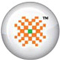PRO DATA DOCTOR it | es | pt | fr | de | jp | kr | cn | ru | nl | gr

Logical Operators

Logical operators of C let you combine two or more relational expressions into a single expression that evaluates to either true or false. Logical operators evaluate to either true or false, depending on the true or false value of their operands.

If x is an integer variable, expressions using logical operators could be written in the following ways:

(x > 1) && (x < 5)
(x >= 2) && (x <= 4)

 Operator Symbol Description Example Assignment operators equal = assign the value of y to x x = y Mathematical operators Increment ++ Increments the operand by one ++x, x++ Decrement -- Decrements the operand by one --x, x-- Addition + Adds two operands x + y Subtraction - Subtracts the second operand from the first x - y Multiplication * Multiplies two operands x * y Division / Divides the first operand by the second operand x / y Modulus % Gives the remainder when the first operand is divided by the second operand x % y Relational operators Equal = = Equality x = = y Greater than > Greater than x > y Less than < Less than x < y Greater than or equal to >= Greater than or equal to x >= y Less than or equal to <= Less than or equal to x <= y Not equal != Not equal to x != y Logical operators AND && True (1) only if both exp1 and exp2 are true; false (0) otherwise exp1 && exp2 OR || True (1) if either exp1 or exp2 is true; false (0) only if both are false exp1 || exp2 NOT ! False (0) if exp1 is true; true (1) if exp1 is false !exp1

Things to remember about logical expressions

 x * = y y - = z + 1 a / = b x + = y / 8 y % = 3 is same as is same as is same as is same as is same as x = x * y y = y - z + 1 a = a / b x = x + y / 8 y = y % 3

Sample Chapters from book DATA RECOVERY WITH AND WITHOUT PROGRAMMING by Author Tarun Tyagi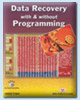Publishers of the Book Number of Pages ISBN Price of the Book BPB Publications, New Delhi, India 540 81-7656-922-4 \$69.00 (Including Shipping Charges, Cost of Book and Other expenses, Free Source Code CD included with the Book)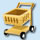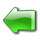page 1 | 2 | 3 | 4 | 5 | 6 | 7 | 8 | 9 | 10 | 11 | 12 | 13 | 14 | 15 | 16 | 17 | 18 | 19 | 20

 page 21 | 22 | 23 | 24 | 25 | 26 | 27 | 28 | 29 | 30 | 31 | 32 | 33 | 34 | 35 | 36 | 37

 page 38 | 39 | 40 | 41 | 42 | 43 | 44 | 45 | 46 | 47 | 48 | 49 | 50 | 51 | 52 | 53 | 54

 page 55 | 56 | 57 | 58 | 59 | 60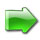© Copyright 2002-2005 DataDoctor.Biz
 Website Data Recovery | recuperación de Datos | Récupération de données | Datenrettung | Recupero dati | データ復旧 | 데이터 복구 | 数据恢复 | Восстановление данных | De terugwinning van gegevens | Ανάκτηση δεδομένων Sitemap Site map1 2 3 4 | Spanish1 2 3 | French1 2 3 | German1 2 3 | Italian1 2 3 | Portuguese1 2 3 | Japanese1 2 3 | Korean1 2 3 | Chinese1 2 3 | Russian1 2 3 | Dutch1 2 3 | Greek1 2 3 Data Recovery Book English | Spanish | French | German | Italian | Portuguese | Japanese | Korean | Chinese | Russian | Dutch | Greek